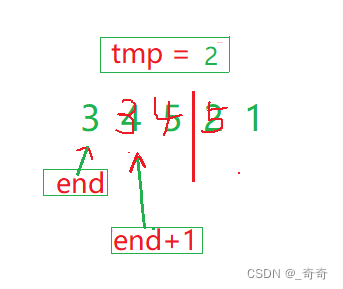﻿ C语言植物大战数据结构希尔排序算法_C 语言_脚本之家

# C语言植物大战数据结构希尔排序算法

更新时间：2022年05月10日 18:02:56   作者：_奇奇

“至若春和景明，波澜不惊，上下天光，一碧万顷，沙鸥翔集，锦鳞游泳，岸芷汀兰，郁郁青青。”

C语言朱武大战数据结构专栏

C语言植物大战数据结构快速排序图文示例

C语言植物大战数据结构堆排序图文示例

C语言植物大战数据结构二叉树递归

## 一、插入排序

5 4 3 2 1

```void InsertSort(int* a, int n)
{
//排序代码
}
int main()
{
int arr[] = { 5,4,3,2,1 };
int sz = sizeof(arr) / sizeof(arr);
InsertSort(arr, sz);
return 0;
}
```

### 1.排序思路

1.第一趟：从下标为1的位置依次往后开始，先让5,4为升序

2.第二趟：来到下标为2的位置，让5,4,3为升序

3.第三趟：来到下标为3的位置，让5,4,3,2为升序

4.第四趟：来到下标为3的位置，让5,4,3,2,1为升序

### 2.单趟排序

1.起始状态。因为能走到第三趟排序，说明第一趟和第二趟已经排好序了，以红色线分割这时的数据为3 4 5 2 1

2.定义指针end指向有序序列的最后一个数，让tmp保存end+1位置的值。(这样可以防止end+1指向的值被覆盖后找不到)3.开始打擂台赛。比较tmp的值和end的值的大小，然后end–。把比tmp大的值依次往后挪动。直到 end < 0越界 或者 tmp比end指向的值大时，单趟排序才完成。

#### 详细图解

(1). tmp为 2 小于 5, 执行a[end+ 1] = a[end];把end+1上的值覆盖。end = end - 1。(2). 2小于 4，继续重复以上步骤。(3).2小于 4，继续重复以上步骤。(4).end此时越界。直接跳出循环，将tmp赋值给a[end + 1];### 3.整体代码

```#include <stdio.h>
void InsertSort(int* a, int n)
{
int i = 0;
//总共n-1躺排序
for (i = 0; i < n - 1; i++)
{
//单趟排序
int end = i;
int tmp = a[end + 1];
while (end >= 0)
{
//打擂台赛
if (tmp < a[end])
{
a[end + 1] = a[end];
end = end - 1;
}
else
{
break;
}
}
a[end + 1] = tmp;
}
}
int main()
{
int arr[] = { 5,4,3,2,1 };
int sz = sizeof(arr) / sizeof(arr);
InsertSort(arr, sz);
return 0;
}
```

### 4.时间复杂度

#### (1).最坏情况下

2.第1趟比较1次，第2趟比较2次，第3躺比较3次。第n-1躺排n-1次。

3.由 等差数列 前n项和公式 (首项 + 末项) * 项数 / 2 得。

T(n) = (n-1)*(1 + n - 1)/2

T(n) = (n-1)*n / 2

4.由大O渐进法表示为O(N2)。

### 5.算法特点

1.是稳定排序

2.代码简单，易实现

3.在数据接近有序 或者 已经有序的情况下，时间复杂度为O(N)

## 二、希尔排序

9,8,7,6,5,4,3,2,1

```void ShellSort(int* a, int n)
{
//排序代码
}
int main()
{
int arr[] = { 9,1,2,5,7,4,1,6,3,5 };
int sz = sizeof(arr) / sizeof(arr);
ShellSort(arr, sz);
return 0;
}
```

### 1.希尔从哪个方面优化的插入排序？

1.因为插入排序有2个特性：当待排序的个数基本有序 和 数据个数较少 时 插入效率较高。

2.所以希尔排序基于这2个特性，先依据间隔(gap)对数据分成各个小组，然后对各组进行预排序，使得数据基本有序，最后再进行一次普通的插入排序使数据整体有序。

### 2.排序思路

1.算法先将要排序的一组数按某个增量gap分成若干组，每组中记录的下标相差gap.

2.对每组中全部元素进行预排序，然后再用一个较小的增量对它进行分组，在每组中再进行预排序。

3.当增量 等于1 时，整个要排序的数被分成一组，排序完成。(这时候相当于 普通的插入排序 了)

### 3.预排序

9,1,2,5,7,4,1,6,3,5

1.间隔(gap)每次一半一半的分。直到最后gap == 1.

2.这样分其实每次都是折半的，常识来说：折半的时间复杂度都为O(LogN).

```#include <stdio.h>
//希尔排序
void ShellSort(int* a, int n)
{
//预排序
int gap = n;
while (gap > 1)
{
//每次gap折半
gap = gap / 2;
}
}
int main()
{
int arr[] = { 9,8,7,6,5,4,3,2,1 };
int sz = sizeof(arr) / sizeof(arr);
ShellSort(arr, sz);
return 0;
}
```

1.gap == 5的分一组进行间隔为5的插入排序。2.gap == 2的分一组，进行间隔(gap)为2的插入排序### 4.正式排序### 5.整体代码```#include <stdio.h>
//希尔排序
void ShellSort(int* a, int n)
{
//预排序
int gap = n;
while (gap > 1)
{
gap = gap / 2;
//进行间隔为gap的插入排序
int i = 0;
for (i = 0; i < n - gap; i++)
{
//单趟排序
int end = i;
int tmp = a[end + gap];
while (end >= 0)
{
if (tmp < a[end])
{
a[end + gap] = a[end];
end = end - gap;
}
else
{
break;
}
}
a[end + gap] = tmp;
}
}
}
int main()
{
int arr[] = { 9,8,7,6,5,4,3,2,1 };
int sz = sizeof(arr) / sizeof(arr);
ShellSort(arr, sz);
return 0;
}
```

### 6.时间复杂度

#### (1).while循环的复杂度

1.假如有n个数据。n一直初以2.最后要等于1.所以公式为n / 2 / 2 / 2 / 2 / 2… = 1.

2.所以设需要初以 x 个2。也就是要执行 x 次。所以n = 2x.

3.每次gap减半的 ，也就是while循环的 时间复杂度就是Log2N

```	int gap = n;
while (gap > 1)
{
//每次gap折半
gap = gap / 2;
}
```

#### (2).每组gap的时间复杂度

1.gap很大很大时，数据跳的很快，差不多时间复杂度为O(N).正式排序时，gap == 1.

2.gap很小，因为数据接近有序，所以时间复杂度也差不多是O(N)